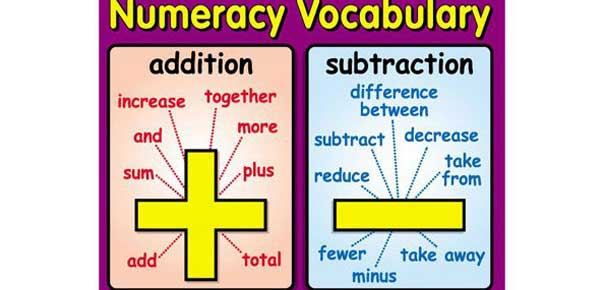# Unit 4 Math Vocabulary Quiz

Approved & Edited by ProProfs Editorial Team
The editorial team at ProProfs Quizzes consists of a select group of subject experts, trivia writers, and quiz masters who have authored over 10,000 quizzes taken by more than 100 million users. This team includes our in-house seasoned quiz moderators and subject matter experts. Our editorial experts, spread across the world, are rigorously trained using our comprehensive guidelines to ensure that you receive the highest quality quizzes.
| By Fizer1993
F
Fizer1993
Community Contributor
Quizzes Created: 1 | Total Attempts: 108
Questions: 13 | Attempts: 112SettingsThere is a lot of terminology involved in the study of mathematic, regardless of what category you’re in. Heck, even simple terms like addition and subtraction have countless synonyms. How much of the verbiage do you know? Let’s find out.

• 1.

### A number that is written using both a whole number and a fraction.

• A.

Common denominator

• B.

Common factor

• C.

Mixed number

• D.

Equivalent fractions

C. Mixed number
Explanation
A mixed number is a number that is written using both a whole number and a fraction. It is a combination of a whole number and a proper fraction. For example, 3 1/2 is a mixed number, where 3 is the whole number and 1/2 is the fraction.

Rate this question:

• 2.

### The product of the denominators of two or more fractions.

• A.

Quick common denominator

• B.

Mixed number

• C.

Common demoninator

• D.

Common factor

A. Quick common denominator
Explanation
A quick common denominator refers to finding the least common multiple of the denominators of two or more fractions in a fast and efficient manner. This allows for easier addition, subtraction, or comparison of the fractions. It is a method used to simplify calculations involving fractions by finding a common denominator quickly. It is different from a common denominator, which is the shared multiple of the denominators of two or more fractions. A mixed number refers to a whole number combined with a fraction, while a common factor refers to a number that divides evenly into two or more numbers.

Rate this question:

• 3.

• 4.

### The price of an Xbox game is normally \$59.99. I bought the game for \$39.99. What did I get on the game?

• A.

Percent

• B.

Discount

• C.

Greatest common factor

• D.

Common denominator

B. Discount
Explanation
The correct answer is "discount" because the question mentions that the normal price of the Xbox game is \$59.99, but the person bought it for \$39.99. The difference between the normal price and the price they paid is \$20.00, which represents the discount they received on the game.

Rate this question:

• 5.

### Which is an example of a common denominator?

• A.

3/7,4/21

• B.

1/2,2/7

• C.

21/30,30/25

• D.

17/25,18/79

A. 3/7,4/21
Explanation
A common denominator is a number that can be divided evenly by the denominators of two or more fractions. In this case, the fractions 3/7 and 4/21 have a common denominator of 21 because both denominators can evenly divide into 21. Therefore, 3/7 and 4/21 are an example of a common denominator.

Rate this question:

• 6.

• 7.

### A counting number of two or more counting numbers is a factor of each of those numbers.

• A.

Equivalent fraction

• B.

Least common multiple

• C.

Common factor

• D.

Mixed number

C. Common factor
Explanation
A common factor is a number that divides evenly into two or more numbers. In this case, the statement is saying that if you have two or more counting numbers, then any counting number that can divide evenly into all of them is a common factor of those numbers. This means that the common factor is a factor of each of the numbers, making it the correct answer.

Rate this question:

• 8.

### Which is an example of equivalent fractions?

• A.

11/22 = 22/44

• B.

10/20 = 15/25

• C.

7/8 = 14/24

• D.

2/3 = 4/5

A. 11/22 = 22/44
Explanation
The given fractions 11/22 and 22/44 are equivalent because they represent the same value. When we simplify the fraction 22/44 by dividing both the numerator and denominator by 2, we get 11/22, which is the same as the first fraction. Therefore, 11/22 = 22/44 is an example of equivalent fractions.

Rate this question:

• 9.

• 10.

### Which is a percent?

• A.

0.73

• B.

73/100

• C.

73

• D.

73%

D. 73%
Explanation
The correct answer is 73% because it represents a value as a percentage. The other options are either decimals or fractions, but 73% is the only one that explicitly states a value as a percentage.

Rate this question:

• 11.

### The smallest number that is a multiple of two or more numbers.

• A.

Least common multiple

• B.

Least common denominator

• C.

Quick common denominator

• D.

Common denominator

A. Least common multiple
Explanation
The least common multiple is the smallest number that is divisible by two or more numbers without leaving a remainder. It is found by identifying the common factors of the given numbers and multiplying them together. As it is the smallest multiple that all the numbers have in common, it is the correct answer for the given question.

Rate this question:

• 12.

• 13.

### Which below is an example of simplest form?

• A.

2/4 = 1/2

• B.

50/100 = 25/50

• C.

30/60 = 15/30

A. 2/4 = 1/2
Explanation
The given example of 2/4 = 1/2 is an example of the simplest form because both fractions have the same numerator and denominator, which means they are equivalent. By dividing both the numerator and denominator by their greatest common divisor, we can simplify the fraction to its lowest terms, which is 1/2.

Rate this question:

Related TopicsBack to top